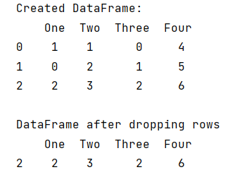# Dropping a row in pandas DataFrame if any value in row becomes 0

Given a Pandas DataFrame, we have to drop a row if any value in row becomes 0. By Pranit Sharma Last updated : September 23, 2023

Pandas is a special tool that allows us to perform complex manipulations of data effectively and efficiently. Inside pandas, we mainly deal with a dataset in the form of DataFrame. DataFrames are 2-dimensional data structures in pandas. DataFrames consist of rows, columns, and the data. The Data inside the DataFrame can be of any type.

Rows in pandas are the different cell (column) values that are aligned horizontally and also provide uniformity. Each row can have the same or different value. Rows are generally marked with the index number but in pandas, we can also assign index names according to the needs. In pandas, we can create, read, update and delete a column or row value.

## Problem statement

Given a Pandas DataFrame, we have to drop a row if any value in row becomes 0.

## Dropping a row in pandas DataFrame if any value in row becomes 0

For this purpose, we will use the ~ invert operator which is used as a not operator and we will select only those values which are not 0 from that row.

Note

To work with pandas, we need to import pandas package first, below is the syntax:

```import pandas as pd
```

Let us understand with the help of an example,

## Python code to drop a row in pandas DataFrame if any value in row becomes 0

```# Importing pandas package
import pandas as pd

# Importing numpy package
import numpy as np

# Creating a dictionary
d = {
'One':[1,0,2],
'Two':[1,2,3],
'Three':[0,1,2],
'Four':[4,5,6]
}

# Creating  dataframe
df = pd.DataFrame(d)

# Display DataFrame
print("Created DataFrame:\n",df,"\n")

# Removing a row if any value becomes 0
df = df[~(df == 0).any(axis=1)]

# Display result
print("DataFrame after dropping rows\n",df)
```

### Output

The output of the above program is: